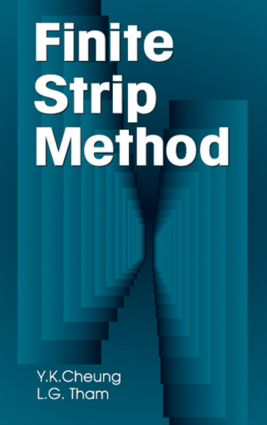# The Finite Strip Method

## 1st Edition

CRC Press

400 pages

Hardback: 9780849374302
pub: 1997-12-29
\$250.00
x

FREE Standard Shipping!

### Description

The increase in the popularity and the number of potential applications of the finite strip method has created a demand for a definitive text/reference on the subject. Fulfilling this demand, The Finite Strip Method provides practicing engineers, researchers, and students with a comprehensive introduction and theoretical development, and a complete treatment of current practical applications of the method.

Written by experts who are arguably the world's leading authorities in the field, The Finite Strip Method covers both the classical strip and the newly developed spline strip and computed shape function strip. Applications in structural engineering, with particular focus on practical structures such as slab-beam bridges, box girder bridges, and tall buildings are discussed extensively. Applications in geotechnology are also covered, as are recently formulated applications in nonlinear analysis.

The Finite Strip Method is a unique book, supplying much-needed information by well-known and highly regarded authors.

Introduction

Interpolation Shape Functions

Choice of Interpolation Shape Functions

Available Interpolation Functions for Classical Finite Strips

Longitudinal Functions for Spline Finite Strips

Computed Shape Function Strips

Constant Strain Conditions

Plate Bending Problems

Introduction

Formulation for Thin Plate Bending

Classical Plate Strips

Spline Bending Strips

Computed Shape Function (COMSFUN) Strips

Curved Plate Strips

Skew Plates

Analysis of Moderately Thick Plates

Least Square Formulation of Plate Bending Problems

Plane Stress Analysis

Introduction

Classical Plane Stress Strips

Spline Plane Stress Strips

Computed Shape Function Strip (COMSFUN)

Curved Plane Stress Strips

Examples

Mixed Formulation of Plane Stress Analysis

Vibration and Stability

Matrix Theory of Free Vibration

Derivation of Consistent Mass Matrix of Classical Strips

Derivation of Consistent Mass Matrix of Spline Strip

Examples

Linear Instability and Geometric (Stress) Stiffness Matrix

Vibration Analysis of Moderately Thick Plates

Modelling of Three Dimensional Solids: Finite Prism and Finite Layer Methods

Introduction

Finite Prism Method

Finite Layer Method

Domain Transformation: Treatments for Arbitrarily Shaped Structures

Introduction

Parametric Representation of Arbitrary Plane Curves

Transformation for Arbitrarily Shaped Quadrilateral Plates

Transformation of Arbitrarily Shaped Shells

Transformation for Longitudinally Arbitrarily Curved Box Girders

Applications to Shell Structures and Bridges

Shell Structures

Bridges

Applications to Tall Buildings

General Consideration and Structural Idealization

Strip Elements

Line Elements

Discrete Beam Model

Examples

Applications to Layered Systems in Structural and Geotechnical Engineering

Sandwich and Laminated Plates

Geotechnical Problems

Non-Linear Analysis

Introduction

Solution for Non-Linear System

Material Non-Linearity

Geometric Non-Linearity

Non-Linear Vibration

Transformation Approach: Fourier and Hankel Transforms

Introduction

Plane Strain Analysis

Three-Dimensional Problem

Viscoelastic Material (Creep)

Consolidation Analysis

Transport Problems

Appendix: Program for the Analysis of Folded Plates and Box Girder Bridges by Classical Finite Strip

Index